Rotational Symmetry

Chapter 14 Class 7 Symmetry
Concept wise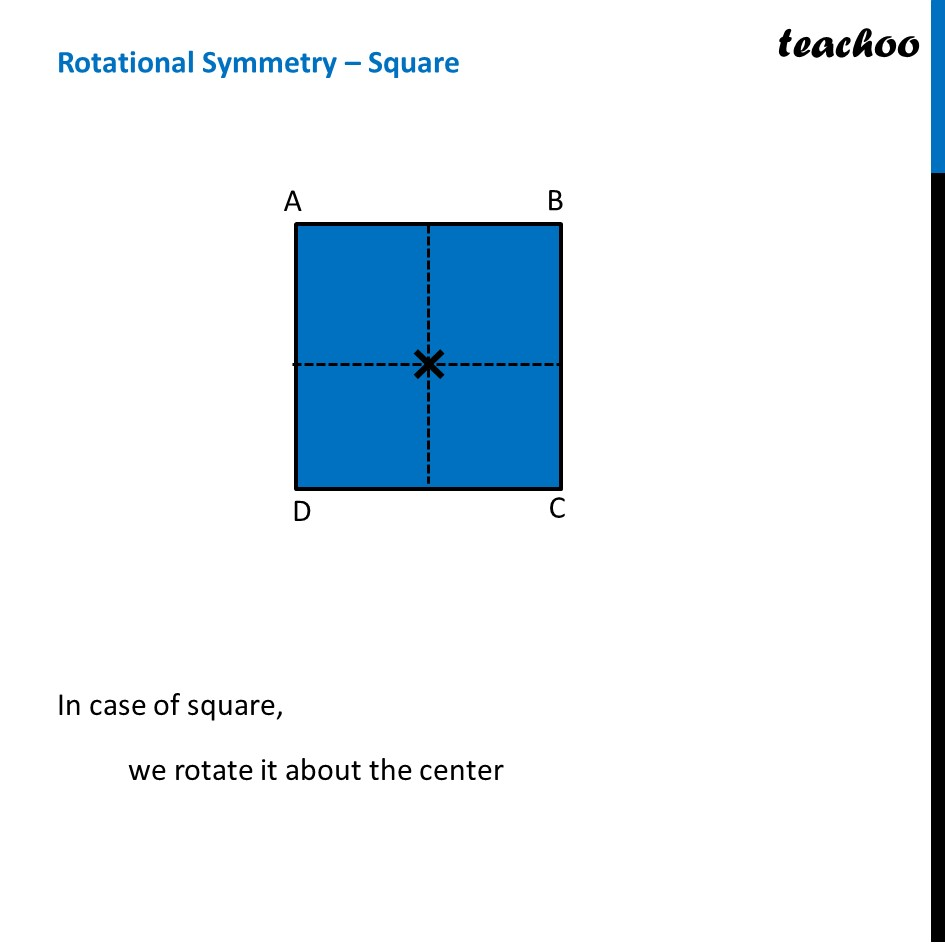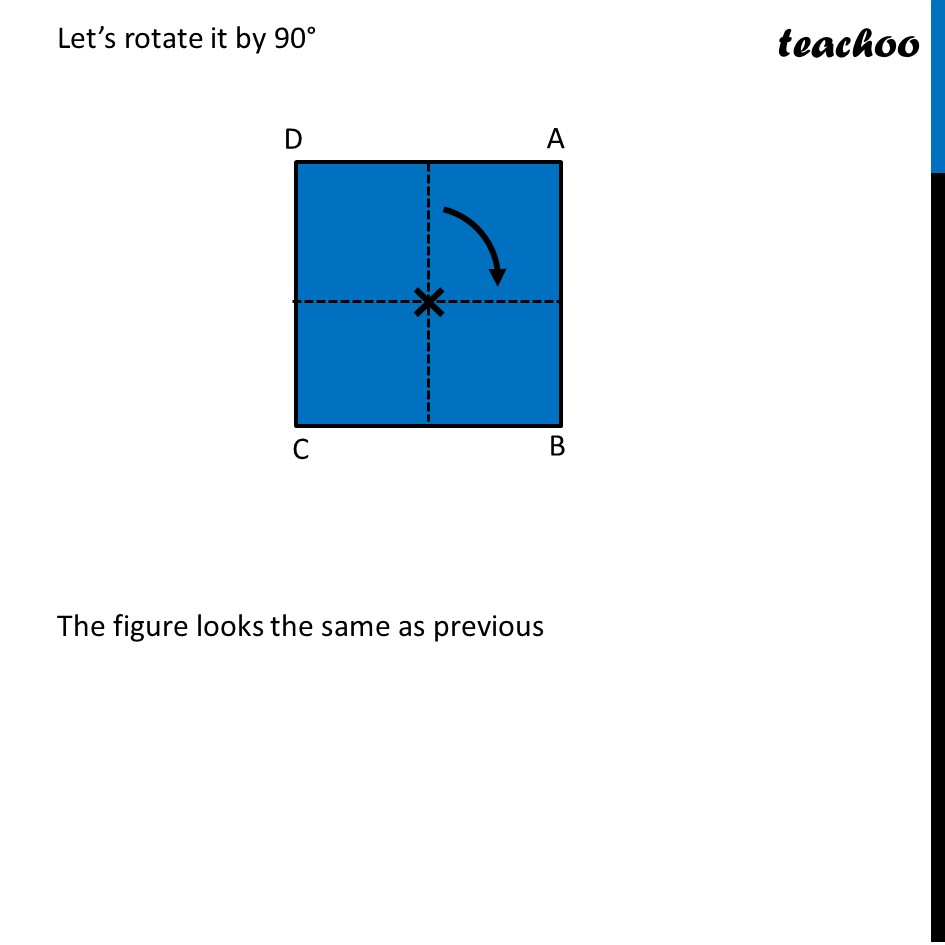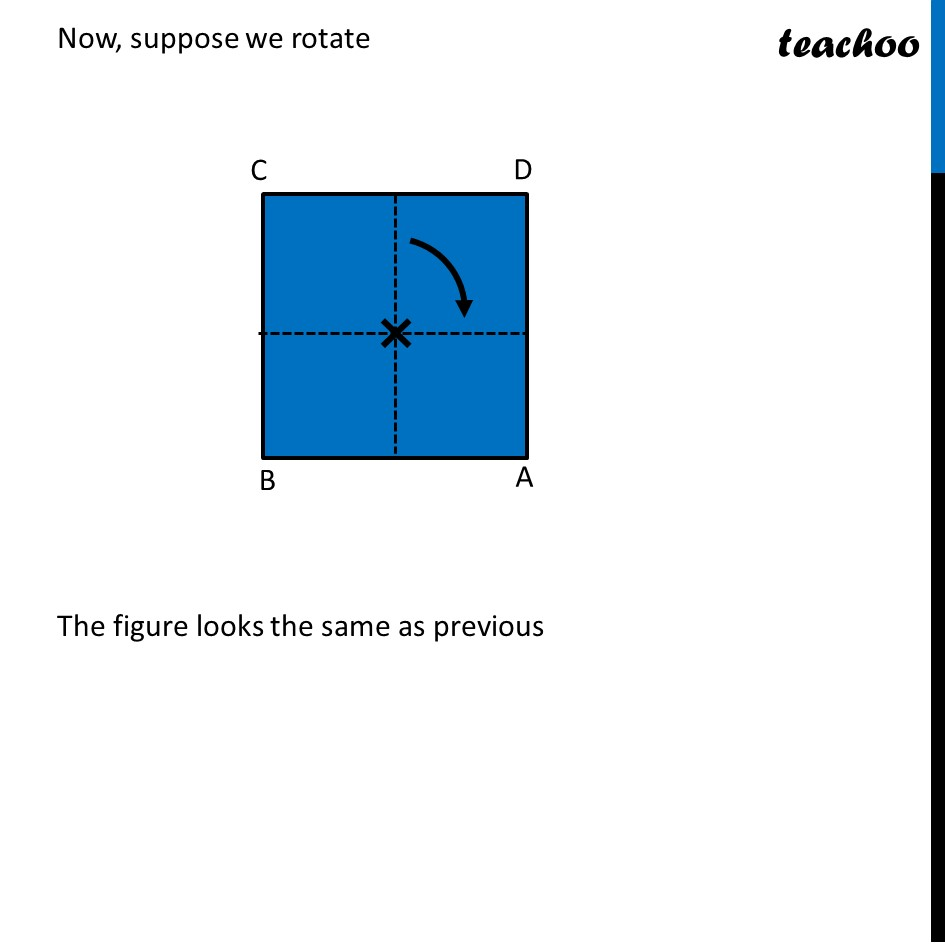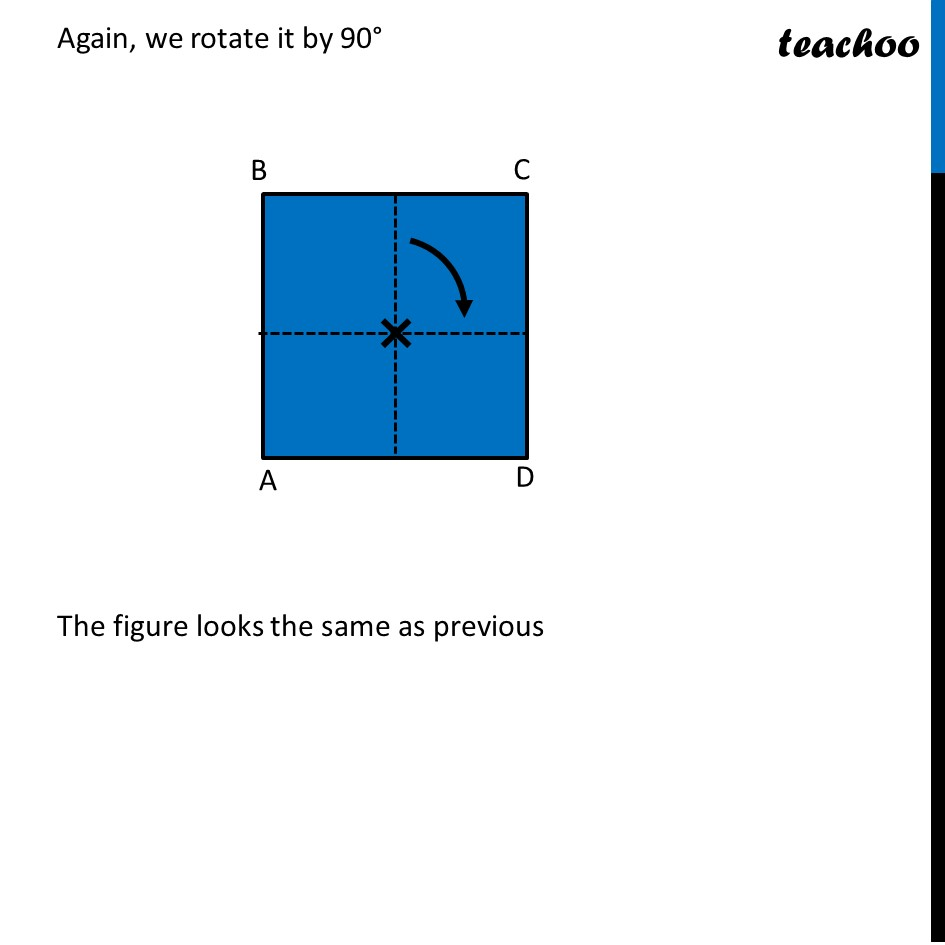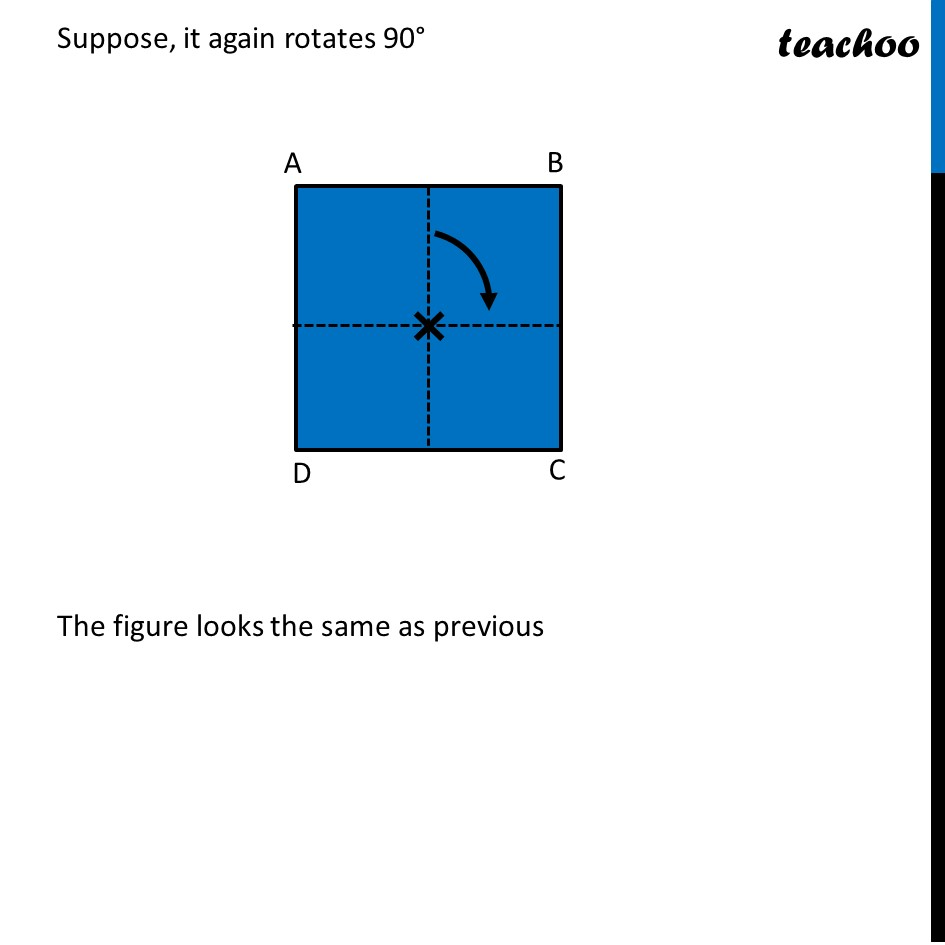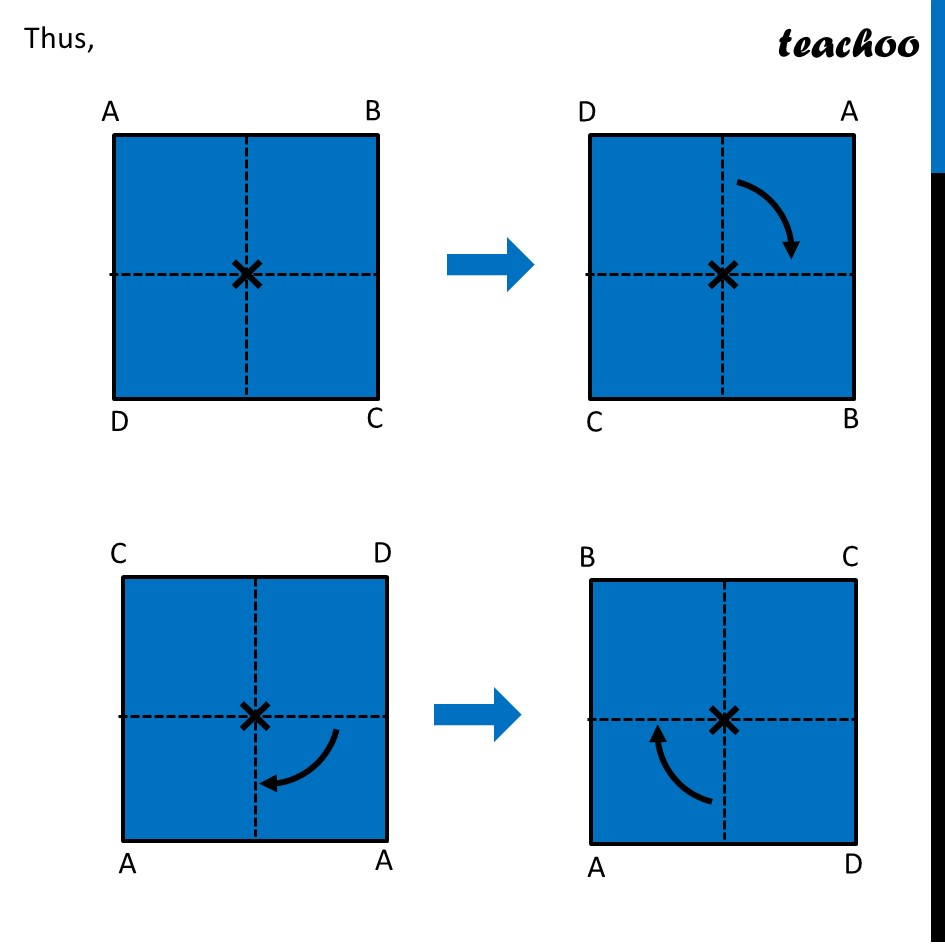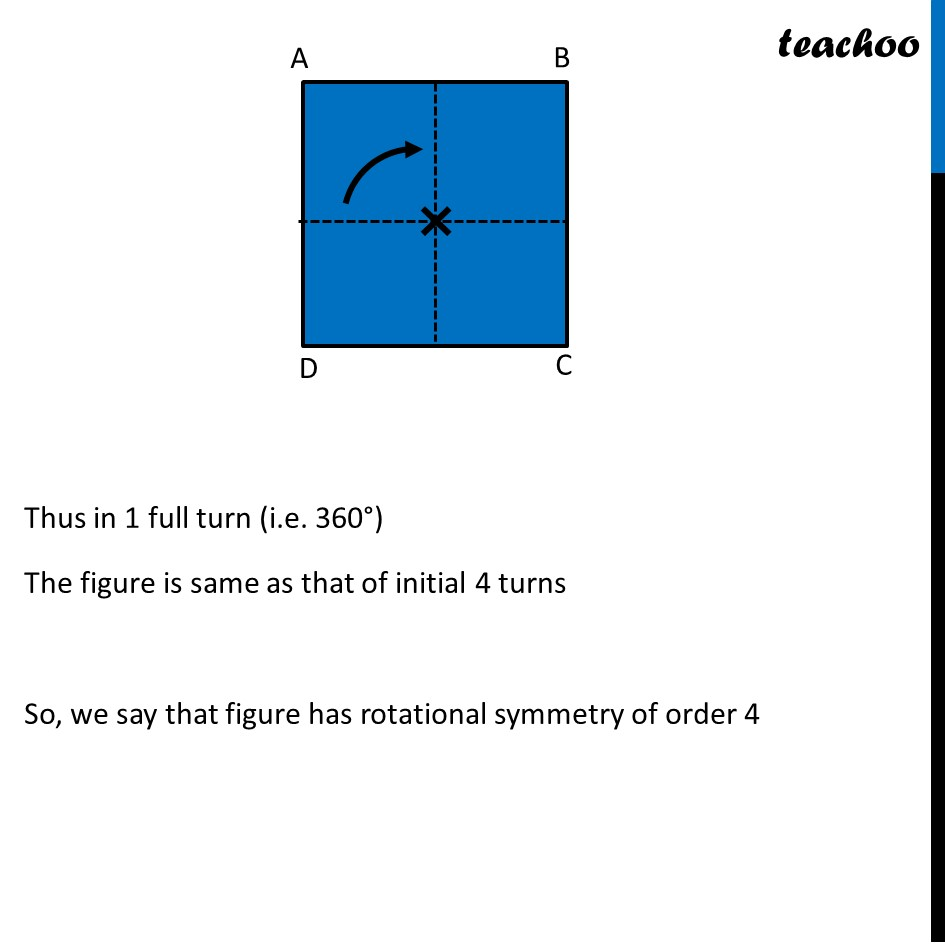Get live Maths 1-on-1 Classs - Class 6 to 12

### Transcript

Rotational Symmetry – Square In case of square, we rotate it about the center Let’s rotate it by 90° The figure looks the same as previous Now, suppose we rotate The figure looks the same as previous Again, we rotate it by 90° The figure looks the same as previous Suppose, it again rotates 90° The figure looks the same as previous Thus, Thus in 1 full turn (i.e. 360°) The figure is same as that of initial 4 turns So, we say that figure has rotational symmetry of order 4